×

Search anything:

# Freivalds’ algorithm for verifying Matrix Multiplication

#### matrix multiplication Algorithms freivalds algorithm Machine Learning (ML)Indian Technical Authorship Contest starts on 1st July 2023. Stay tuned.

Reading time: 30 minutes | Coding time: 12 minutes

Freivalds' algorithm is a probabilistic randomized algorithm used to verify matrix multiplication. Given three n x n matrices, Freivalds' algorithm determines in O(kn^2) whether the matrices are equal for a chosen k value with a probability of failure less than 2^-k.

### Freivalds' algorithm

1. Input: n×n matrices A, B and C.

2. We would like to verify if A×B=C.
Choose a n×1 column vector r→∈{0,1}n, uniformly and at random. In other words, each component of the vector is either 0 or 1 and chosen randomly and uniformly.

3. Compute A⋅(B⋅r) and C⋅r. This takes Θ(n^2) time.

4. Now comes the output

• If A⋅(B⋅r)≠C⋅r, output FALSE. In other words, we say that the given matrix multiplication is incorrect. This has a probability of correctness = 1.
• If A⋅(B⋅r)=C⋅r, output TRUE. In other words, we say that the given matrix multiplication is correct. This has a probability of correctness ≥1/2.

### Example

Let A,B,C are the matrices such that A × B = C.A random two-element vector with entries equal to 0 or 1 is selected Vector r=[1,1] .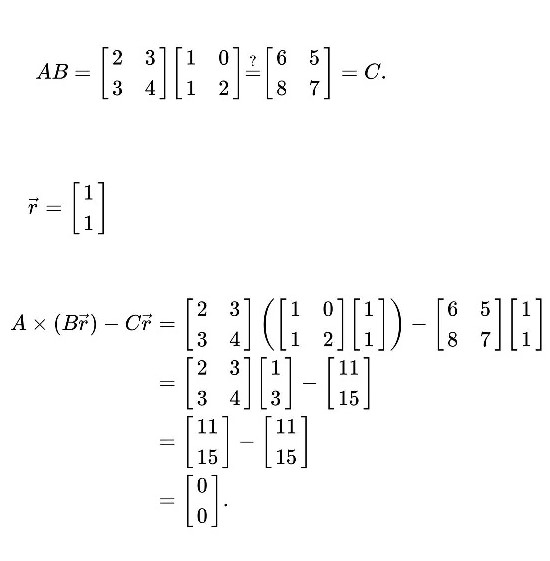This yields the zero vector, suggesting the possibility that AB = C. However, if in a second trial the vector r=[1,0] is selected, the result becomes: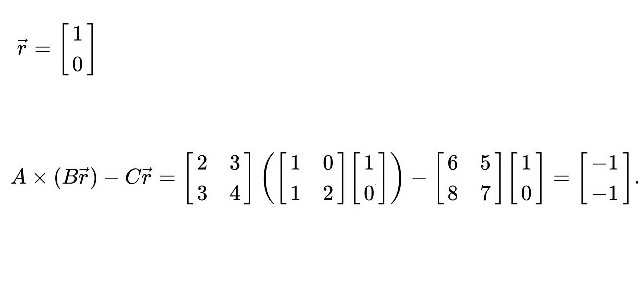The result is nonzero, proving that in fact AB ≠ C.
There are four two-element 0/1 vectors, and half of them give the zero vector in this case Vector r=[0,0] and r=[1,1].so the chance of randomly selecting these in two trials (and falsely concluding that AB=C) is 1/22 or 1/4. In the general case, the proportion of r yielding the zero vector may be less than 1/2, and a larger number of trials would be used, rendering the probability of error very small.

### Error analysis

Let p equal the probability of error. We claim that if A × B = C, then p = 0, and if A × B ≠ C, then p ≤ 1/2.

Case A × B = C:
Regardless of the value of vector r, since it uses only that A × B - C =0. Hence the probability for error in this case would be zero.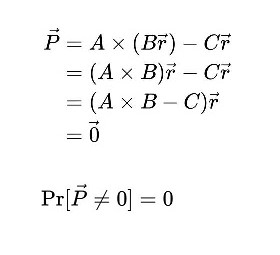Case A × B ≠ C: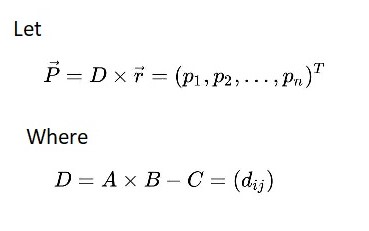Since A × B ≠ C, we have that some element of D is nonzero. Suppose that the element d≠0.For some constant y. Using Bayes' Theorem, we can partition over y.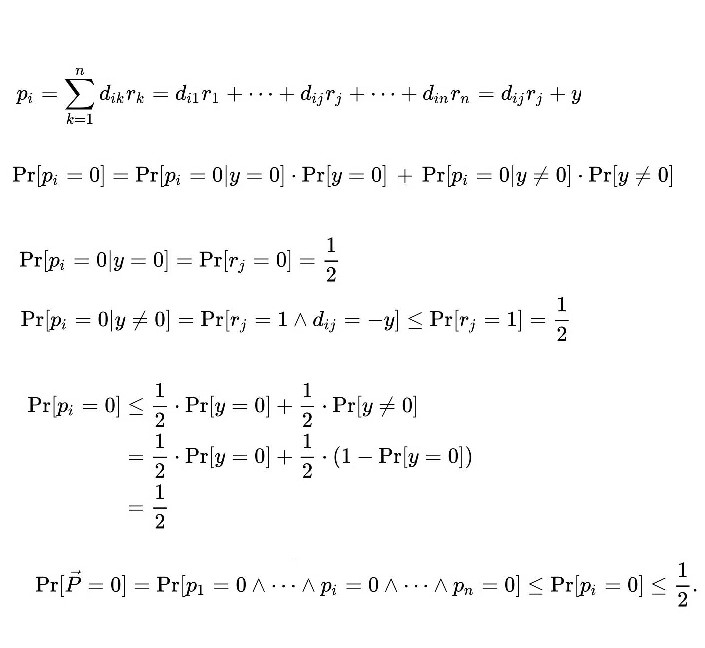### Pseudocode

• Generate an n × 1 random 0/1 vector r.
• Compute P = A × (Br) – Cr.
• Return true if P = ( 0, 0, …, 0 )T, return false otherwise.

Br = matrix B multiplied by Vector r.
Cr = matrix C multiplied by Vector r.

### Complexity

• Worst case time complexity: `Θ(kn^2)`

• Space complexity: `Θ(n^2)`
k = number of times the algorithm iterates.

• C++

### C++

``````
#include <iostream>
#include <stdio.h>
#include <stdlib.h>
using namespace std;
int main(int argc, char **argv)
{
cout << "Enter the dimension of the matrices: " << endl;
int n;
cin >> n;
cout << "Enter the 1st matrix: " << endl;
double a[n][n];
for (int i = 0; i < n; i++)
{
for (int j = 0; j < n; j++)
{
cin >> a[i][j];
}
}
cout << "Enter the 2nd matrix: " << endl;
double b[n][n];
for (int i = 0; i < n; i++)
{
for (int j = 0; j < n; j++)
{
cin >> b[i][j];
}
}
cout << "Enter the result matrix: " << endl;
double c[n][n];
for (int i = 0; i < n; i++)
{
for (int j = 0; j < n; j++)
{
cin >> c[i][j];
}
}
//random generation of the r vector
// containing only 0/1 as its elements
double r[n];
for (int i = 0; i < n; i++)
{
r[i] = rand() % 2;
}
//test A * (b*r) - (C*) = 0
double br[n];
for (int i = 0; i < n; i++)
{
for (int j = 0; j < 1; j++)
{
for (int k = 0; k < n; k++)
{
br[i][j] = br[i][j] + b[i][k] * r[k][j];
}
}
}
double cr[n];
for (int i = 0; i < n; i++)
{
for (int j = 0; j < 1; j++)
{
for (int k = 0; k < n; k++)
{
cr[i][j] = cr[i][j] + c[i][k] * r[k][j];
}
}
}
double abr[n];
for (int i = 0; i < n; i++)
{
for (int j = 0; j < 1; j++)
{
for (int k = 0; k < n; k++)
{
abr[i][j] = abr[i][j] + a[i][k] * br[k][j];
}
}
}
//    br = multiplyVector(b, r, n);
//    cr = multiplyVector(c, r, n);
//    abr = multiplyVector(a, br, n);
//abr-cr
for (int i = 0; i < n; i++)
{
abr[i] -= cr[i];
}
bool flag = true;
for (int i = 0; i < n; i++)
{
if (abr[i] == 0)
continue;
else
flag = false;
}
if (flag == true)
cout << "True";
else
cout << "False";
}

``````

### Applications

• Fingerprinting is a well known technique, which is often used in designing Monte Carlo algorithms for verifying identities involving matrices, integers and polynomials.

• Freivalds' algorithm frequently arises in introductions to probabilistic algorithms due to its simplicity and how it illustrates the superiority of probabilistic algorithms in practice for some problems.#### Kyatham Srikanth

Software Developer at Optum | Summer Research Intern at Central Institute Of Mining & Fuel Research (2017) and OpenGenus (2018) | B. Tech (2019) at Indian Institute of Technology (IIT BHU), Varanasi